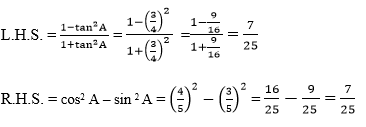Newbie

# If 3 cot A = 4, check whether (1-tan2 A)/(1+tan2 A) = cos2 A – sin 2 A or not. Q.8

• 0

How i solve the ncert class 10 introduction of trigonometry question of exercise 8.1 question no.8 , this question is  very hard to solve . Sir please help me to solve this question because  i can’t  solve this question properly .If 3 cot A = 4, check whether (1-tan2 A)/(1+tan2 A) = cos2 A – sin 2 A or not.

Share

1. Let △ABC in which ∠B=90°

We know that, cot function is the reciprocal of tan function and it is written as

cot(A) = AB/BC = 4/3

Let AB = 4k an BC =3k, where k is a positive real number.

According to the Pythagorean theorem,

AC2=AB2+BC2

AC2=(4k)2+(3k)2

AC2=16k2+9k2

AC2=25k2

AC=5k

Now, apply the values corresponding to the ratios

tan(A) = BC/AB = 3/4

sin (A) = BC/AC = 3/5

cos (A) = AB/AC = 4/5

Now compare the left hand side(LHS) with right hand side(RHS)Since, both the LHS and RHS = 7/25

R.H.S. =L.H.S.

Hence, (1-tan2 A)/(1+tan2 A) = cos2 A – sin 2 A  is proved

• 0